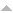# Publications

Found 248 results
Author Title [ Type] Year
Filters: First Letter Of Last Name is Y  [Clear All Filters]
Articles in international peer reviewed journals
Compositional structure of integral transformations. Dokl. Akad. Nauk SSSR. 1986;286:786-790.Edit
On the construction of integral transformations by the composition method. Izv. Vyssh. Uchebn. Zaved. Mat.. 1993:71-79 (1994).
A constructive method for constructing integral convolutions. Dokl. Akad. Nauk BSSR. 1990;34:588-591, 666.
On the convolution for the Kontorovich-Lebedev transformation and its applications to integral equations. Dokl. Akad. Nauk BSSR. 1987;31:101-103, 188.
Convolution Hilbert spaces associated with the Kontorovich-Lebedev transformation. Thai J. Math.. 2003;1:9-16.
On convolution integrals associated with \$H\$-transforms. J. Fract. Calc.. 1997;11:53-65.Edit
The convolution method for the development of new Leibniz rules involving fractional derivatives and of their integral analogues. Integral Transform. Spec. Funct.. 1993;1:119-134.Edit
A convolution operator related to the generalized Mehler-Fock and Kontorovich-Lebedev transforms. Results Math.. 2013;63:511-528.Edit
Convolution operators related to the Fourier cosine and Kontorovich-Lebedev transformations. Results Math.. 2009;55:175-197.Edit
A convolution related to the inverse Kontorovich-Lebedev transform. Sarajevo J. Math.. 2007;3(16):215-232.Edit
Convolutions and neutrix convolution in connection with the incomplete gamma function. Rad. Mat.. 2002;11:37-47.Edit
Convolutions for \$H\$-function transformations. Indian J. Pure Appl. Math.. 1992;23:743-752.Edit
Convolutions related to the Fourier and Kontorovich-Lebedev transforms revisited. Integral Transforms Spec. Funct.. 2010;21:259-276.Edit
A criterion for the unitarity of a two-sided integral transformation. Ukraï n. Mat. Zh.. 1992;44:697-699.Edit
On the curious series related to the elliptic integrals. The Ramanujan Journal. 2018;45(3):797-815.
A distribution associated with the Kontorovich-Lebedev transform. Opuscula Math.. 2006;26:161-172.
Donoho-Stark and Paley-Wiener theorems for the \$G\$-transform. Adv. in Appl. Math.. 2010;45:108-124.Edit
A double index transform with a product of Macdonald's functions revisited. Opuscula Math.. 2009;29:313-329.
Eigenfunctions and fundamental solutions of the fractional two-parameter Laplacian. Int. J. Math. Math. Sci.. 2010:Art. ID 541934, 18.
Extremely Abundant Numbers and the Riemann Hypothesis. Journal of Integer Sequences. 2014;17(2):Article 14.2.8.Edit
The Fourier-Stieltjes transform of Minkowski's \$?(x)\$ function and an affirmative answer to Salem's problem. C. R. Math. Acad. Sci. Paris. 2011;349:633-636.
Fundamental solutions of the fractional two-parameter telegraph equation. Integral Transforms Spec. Funct.. 2012;23:509-519.Edit
A general approach to the theory of integral transforms with respect to an index. Izv. Vyssh. Uchebn. Zaved. Mat.. 1986:77-79, 84.
A general class of Voronoi's and Koshliakov-Ramanujan's summation formulas involving \$d_k(n)\$. Integral Transforms Spec. Funct.. 2011;22:801-821.